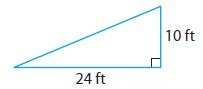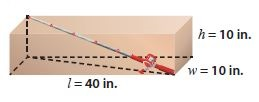Homework Explained - Math Practice 101Dear guest, you are not a registered member. As a guest, you only have read-only access to our books, tests and other practice materials.

As a registered member you can:

Registration is free and doesn't require any type of payment information. Click here to Register.
Go to page:
Chapter 12:The Pythagorean Theorem; Lesson 1: The Pythagorean Theorem

### Guided Practice

• Question 1

Find the length of the missing side of the triangle$$a^ 2 + b ^2 = c^ 2 → 24 ^2 +\color{red}{?}=c^2\rightarrow\color{red}{?}=c^2$$

The length of the hypotenuse is _____ feet.

•  feet
• Question 2

Mr. Woo wants to ship a fishing rod that is 42 inches long to his son. He has a box with the dimensions shown.a. Find the square of the length of the diagonal across the bottom of the box.

•  inches
• Question 2

b. Find the length from a bottom corner to the opposite top corner to the nearest tenth. Will the fishing rod fit?

•  inches

### ESSENTIAL QUESTION CHECK-IN

• Question 3

State the Pythagorean Theorem and tell how you can use it to solve problems.

• Type below:

Yes, email page to my online tutor. (if you didn't add a tutor yet, you can add one here)